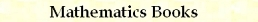Basic Metric C/C++ Macros
by Stanislav Sýkora, Extra Byte, Via R.Sanzio 22C, Castano Primo, Italy 20022
in Stan's Library, Ed.S.Sykora, Vol.II. First release February 15, 2007.
Permalink via DOI:  10.3247/SL2Soft07.001
 PREVIOUS | NEXT | Code snippets | Software Books and Links Programming Section of Stan' LIBRARY | Stan's HUB
 This document is a part of a collection of macros. For introductory remarks, see the first part (General Purpose Macros). For a review of macro-syntax rules and related tips and tricks, see Writing Macros. Typically, the arguments of basic metric macros belong to one of the basic numeric types (for example, float of double), but they could be any types for which the concept of distance coincides with the expression mAbs((b)-(a)), where mAbs is the absolute-value macro defined in Extrema Macros. As explained below, this can be generalized to other types of entities, provided that one (i) redefines in an appropriate way the macro mDist and (ii) systematically changes the names of all the macros. An example is given by the Complex Metric Macros, a file which can be used as a template for other types.
 CATEGORY MACRO DEFINITIONs mNorm, mDist DISTANCE to mDistTo2, mDistTo3, mDistTo4, mDistTo5, mDistTo6 MAX DISTANCE mMaxDistTo2, mMaxDistTo3, mMaxDistTo4, mMaxDistTo5, mMaxDistTo6 NEAREST to mNearest2, mNearest3, mNearest4, mNearest5, mNearest6 MOST REMOTE mRemotest2, mRemotest3, mRemotest4, mRemotest5, mRemotest6 SEPARATIONs mMaxSep2, mMaxSep3, mMaxSep4, mMaxSep5, mMaxSep6 mMinSep2, mMinSep3, mMinSep4, mMinSep5, mMinSep6
 Distance definition Code: #define mNorm(a) mAbs(a) #define mDist(a,b) mNorm((b)-(a)) Arguments: Normally, a and b stand for any numeric-type entities (including expressions) which are compatible with the binary operator - (minus) and for which comparisons with zero are defined and make sense so that the macro mAbs can be used. This includes all basic numeric types of C and C++. However, a and b might also be elements of a more generic metric sets (for example complex numbers, 2D and 3D vectors, etc.). In such a case, mDist must be redefined to return the real-valued distance between its arguments. This can be achieved either indirectly, by redefining the macro mNorm, or by dropping mNorm and providing a new, direct definition of mDist. Once done all the other macros remain valid. The only inconvenience is that, in order to keep the various metric types separate, one needs to copy them and change all their names. If you need to do this, use the Complex Metric Macros as a template. mNorm(a) and mDist(a,b) In their basic form, these macros are equivalent to the mathematical expressions |a| and |b-a|. As explained above, when the arguments are not simple numeric types, but nevertheless elements of a metric space, the macro mDist needs to be redefined to return the real-valued distance between its arguments.
 [Minimum] distance from a set of elements Code: #define mDistTo2(x,a,b) mMin(mDist(x,a),mDist(x,b)) #define mDistTo3(x,a,b,c) mMin(mDist(x,a),mDistTo2(x,b,c)) #define mDistTo4(x,a,b,c,d) mMin(mDistTo2(x,a,b),mDistTo2(x,c,d)) #define mDistTo5(x,a,b,c,d,e) mMin(mDistTo2(x,a,b),mDistTo3(x,c,d,e)) #define mDistTo6(x,a,b,c,d,e,f) mMin(mDistTo3(x,a,b,c),mDistTo3(x,d,e,f)) Arguments: x and a through f are subject to the same requirements as the arguments of the macro mDist discussed above. In particular, they may be expressions. The macros rely on the distance-definition macro mDist and on the relational macro mMin. They are highly optimized using binary set-partitioning. mDistTo2(x,a,b), mDistTo3(x,a,b,c), mDistTo4(x,a,b,c,d), mDistTo5(x,a,b,c,d,e), mDistTo6(x,a,b,c,d,e,f) These macros return the shortest distance between x and the set of elements {a,b,...}.
 Maximum distance from the elements of a set Code: #define mMaxDistTo2(x,a,b) mMax(mDist(x,a),mDist(x,b)) #define mMaxDistTo3(x,a,b,c) mMax(mDist(x,a),mMaxDistTo2(x,b,c)) #define mMaxDistTo4(x,a,b,c,d) mMax(mMaxDistTo2(x,a,b),mMaxDistTo2(x,c,d)) #define mMaxDistTo5(x,a,b,c,d,e) mMax(mMaxDistTo2(x,a,b),mMaxDistTo3(x,c,d,e)) #define mMaxDistTo6(x,a,b,c,d,e,f) mMax(mMaxDistTo3(x,a,b,c),mMaxDistTo3(x,d,e,f)) Arguments: x and a through f are subject to the same requirements as the arguments of the macro mDist discussed above. In particular, they may be expressions. The macros rely on the distance-definition macro mDist and on the relational macro mMax. They are highly optimized using binary set-partitioning. mMaxDistTo(x,a,b), mMaxDistTo2(x,a,b), mMaxDistTo3(x,a,b,c), mMaxDistTo4(x,a,b,c,d), mMaxDistTo5(x,a,b,c,d,e), mMaxDistTo6(x,a,b,c,d,e,f) These macros return the largest distance between x and any element of the set {a,b,...}.
 Value of the nearest set element Code: #define mNearest2(x,a,b) (mIsLT(mDist(x,a),mDist(x,b)) ? (a) : (b)) #define mNearest3(x,a,b,c) mNearest2(x,a,mNearest2(x,b,c)) #define mNearest4(x,a,b,c,d) mNearest2(x,mNearest2(x,a,b),mNearest2(x,c,d)) #define mNearest5(x,a,b,c,d,e) mNearest2(x,mNearest2(x,a,b),mNearest3(x,c,d,e)) #define mNearest6(x,a,b,c,d,e,f) mNearest2(x,mNearest3(x,a,b,c),mNearest3(x,d,e,f)) Arguments: x and a through f are subject to the same requirements as the arguments of the macro mDist discussed above. In particular, they may be expressions. The macros rely on the distance-definition macro mDist and on the relational macro mIsLT. They are optimized using binary set-partitioning. mNearest2(x,a,b), mNearest3(x,a,b,c), mNearest4(x,a,b,c,d), mNearest5(x,a,b,c,d,e), mNearest6(x,a,b,c,d,e,f) These macros return the value of the element of the set {a,b,...} which is nearest to x.
 Value of the most remote set element Code: #define mRemotest2(x,a,b) (mIsGT(mDist(x,a),mDist(x,b)) ? (a) : (b)) #define mRemotest3(x,a,b,c) mRemotest2(x,a,mRemotest2(x,b,c)) #define mRemotest4(x,a,b,c,d) mRemotest2(x,mRemotest2(x,a,b),mRemotest2(x,c,d)) #define mRemotest5(x,a,b,c,d,e) mRemotest2(x,mRemotest2(x,a,b),mRemotest3(x,c,d,e)) #define mRemotest6(x,a,b,c,d,e,f) mRemotest2(x,mRemotest3(x,a,b,c),mRemotest3(x,d,e,f)) Arguments: x and a through f are subject to the same requirements as the arguments of the macro mDist discussed above. In particular, they may be expressions. The macros rely on the distance-definition macro mDist and on the relational macro mIsGT. They are optimized using binary set-partitioning. mRemotest2(x,a,b), mRemotest3(x,a,b,c), mRemotest4(x,a,b,c,d), mRemotest5(x,a,b,c,d,e), mRemotest6(x,a,b,c,d,e,f) These macros return the value of the element of the set {a,b,...} which is most remote from x.
 Maximum and minimum separations between set elements Code: #define mMaxSep2(a,b) mDist(a,b) #define mMaxSep3(a,b,c) mMax(mMaxDistTo2(a,b,c),mMaxSep2(b,c)) #define mMaxSep4(a,b,c,d) mMax(mMaxDistTo3(a,b,c,d),mMaxSep3(b,c,d)) #define mMaxSep5(a,b,c,d,e) mMax(mMaxDistTo4(a,b,c,d,e),mMaxSep4(b,c,d,e)) #define mMaxSep6(a,b,c,d,e,f) mMax(mMaxDistTo5(a,b,c,d,e,f),mMaxSep5(b,c,d,e,f)) #define mMinSep2(a,b) mDist(a,b) #define mMinSep3(a,b,c) mMin(mDistTo2(a,b,c),mMinSep2(b,c)) #define mMinSep4(a,b,c,d) mMin(mDistTo3(a,b,c,d),mMinSep3(b,c,d)) #define mMinSep5(a,b,c,d,e) mMin(mDistTo4(a,b,c,d,e),mMinSep4(b,c,d,e)) #define mMinSep6(a,b,c,d,e,f) mMin(mDistTo5(a,b,c,d,e,f),mMinSep5(b,c,d,e,f)) Arguments: x and a - f are subject to the same requirements as the arguments of the macro mDist discussed above. In particular, they may be expressions. The macros rely on the distance-definition macro mDist and on the relational macros mMax and mMin. They are highly optimized using binary set-partitioning. mMaxSep2(a,b) mMaxSep3(a,b,c) mMaxSep4(a,b,c,d) mMaxSep5(a,b,c,d,e) mMaxSep6(a,b,c,d,e,f) These macros return the maximum distance between any two elements of the set {a,b,...}. In some contexts, this quantity is known as the diameter of the set. mMinSep2(a,b) mMinSep3(a,b,c) mMinSep4(a,b,c,d) mMinSep5(a,b,c,d,e) mMinSep6(a,b,c,d,e,f) These macros return the minimum distance between any two elements of the set {a,b,...}.TOP | PREVIOUS | NEXT | Code snippets | Software Books and Links Programming Section of Stan' LIBRARY | Stan's HUB
 Copyright ©2007 Stanislav Sýkora    DOI: 10.3247/SL2Soft07.001 Designed by Stan Sýkora